# Site map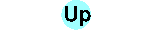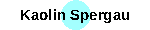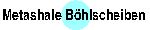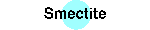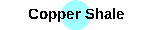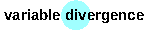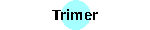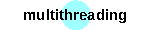# Combined refinement

With enabling BGMN for neutron diffraction, combined refinement of data from at least two experiments (one neutron, the other laboratory X-ray or synchrotron data) becomes of interest. Combined refinement was enabled with BGMN at main level 5.

combined refinement will be switched on by setting `NDEVICES=...` to the number of devices in the BGMN task description `*.sav` file. As a basic principle, combined refinement has multiple wavelength distributions, multiple device functions etc. In following, most of the entries in the *.sav file will be enriched by an additional (leftmost) index for the device number:

`RU``RU[i]`,
`EPS1``EPS1[i]`,
`EPS2``EPS2[i]`,
`EPS3``EPS3[i]`,
`EPS4``EPS4[i]`,
`LAMBDA``LAMBDA[i]`,
`SYNCHROTRON``SYNCHROTRON[i]`,
`NEUTRONS``NEUTRONS[i]`,
`VERZERR``VERZERR[i]`,
`POL``POL[i]`,
`VAL[j]``VAL[i,j]`,
`WMIN``WMIN[i]`,
`WMAX``WMAX[i]`,
`CUT[j]``CUT[i,j]`,
`DIAGRAMM``DIAGRAMM[i]`,
`OUTPUT``OUTPUT[i]` etc.

Note: For the moment, the BGMNwin GUI does not understand the `DIAGRAMM[i]` entries. As a workaround, one may set an additional non-indexed `DIAGRAMM` entry, thus that diagram will be displayed during refinement.

No changes are to the `LIST` and `STRUC[i]` entries. The file as refered by the `LIST` entry will contain results for all devices. Structure description files `*.str` may contain several new items:

• `DeviceSelect(i,...)` may be inserted at any position in the `*.str` file. In following, all subsequent entries in the `*.str` file will be valid only for the selected devices. Note: At least lattice parameters and space group must be unique for one structure. In following `DeviceSelect` will be ignored for lattice parameter and space group detection.
• There is a new keyword for preferred orientation description by `PO=SPHAR2` etc. In difference to `GEWICHT=SPHAR2` etc, `PO` will set a pure preferred orientation model with mean value 1. You must give an additional scalar value for `GEWICHT` (or `GEWICHT`) to scale the calculated to the measured pattern, for that reason. Both `PO` plus `GEWICHT` will be used in combination. This is useful in case of multidevice evaluation of a multiphase sample: The phase's scale factors will be of unique ratio for all the devices. But preferred orientation of a phase will change between devices, in general. In following, the `PO` feature has to be used. Note: `PO=SPHAR...` and `GEWICHT=SPHAR...` are mutual exclusive for a given device. In following, you may not use `GEWICHT=SPHAR0` for defining a scalar value of `GEWICHT`. You must use `PARAM=GEWICHT=0_0` instead.

Attention: In contrast to `GEWICHT=SPHARx`, there is no automatic switch-down for `PO=SPHARx`.

• `idev` refers to the actual device number, `ifxray` is a logical switch which is true in case the actual device is an X-ray one, `ifsynchrotron` is a logical switch which is true in case the actual device is a synchrotron one and `ifneutrons` is a logical switch which is true in case the actual device is a neutrons one.

We are grateful to Dr. H. Boysen (LMU Munich) for kindly supporting the patterns. This example shows some pitfalls of multi device refinement. We will not discuss the geometric device functions here, there is still room for their improvement. In special, for the neutron device (SPODI at FRM II in Munich), a width formula for a single squared lorentzian was used. A minor content of β-PbO (Massicot) was present.

As a first step, the BGMN control file `BleiTitanat.sav` is set up:

```NDEVICES=2
NEUTRONS=0.1548
PI=2*acos(0)
HWB=sqrt(sqr(0.204)+sqr(0.21*sin(THETA*PI/180)*tan((90-zweiTheta)*PI/180)))
VERZERR=HWB*PI/(720*sqrt(sqrt(2)-1))
VAL[1,1]=BleiTitanat_neutronen.dat
WMIN=10
OUTPUT=BleiTitanat_neutronen
DIAGRAMM=BleiTitanat_neutronen
PARAM=EPS1=0_-0.005^0.005
PARAM=EPS2=0_-0.005^0.005
LAMBDA=mo1
VERZERR=device_xray
% Germanium-Monochromator A=5.658 (111)? d=3.267 A
POL=sqr(cos(12.46*PI/180))
VAL[2,1]=BleiTitanat_xray.dat
OUTPUT=BleiTitanat_xray
DIAGRAMM=BleiTitanat_xray
PARAM=EPS1=0_-0.005^0.005
PARAM=EPS2=0_-0.005^0.005
STRUC=PbTiO3.str
STRUC=PbO_beta_Massicot.str
gdev=1
PARAM=gdev=1_0
denom=PbObetaMassicot+PbTiO3
GOAL=PbTiO3/denom
GOAL=PbObetaMassicot/denom
LIST=BleiTitanat
PROTOKOLL=Y
```
As you may see, we have introduced indexed `EPS1`/`EPS2` for both the devices as parameter. A fifth parameter `gdev` controls the principal ratio of intensities between both the devices. We use a named wavelength for the Mo Kα1 radiation, Pb has remarkable f'=-3.39 plus f"=10.11 for Mo Kα.

The next step will be setting up PbTiO3 and PbO structure files for combined refinement.
File `PbTiO3.str`:

```PHASE=PbTiO3_90693  SpacegroupNo=99 Setting=1 HermannMauguin=P4mm //
PARAM=A=0.3904_0.3884^0.3923 PARAM=C=0.4135_0.4114^0.4155 //
RP=4 PARAM=B1=0_0^0.01 PARAM=k1=0_0^1
ANISOLIMIT=0 ANISO4LIMIT=0 k2=ANISO4^0.001
// neutron data have no PO
DeviceSelect(2) PO=SPHAR6 DeviceSelect(1,2)
PARAM=GEWICHT=0_0 GEWICHT=gdev[idev]*GEWICHT*ifthenelse(ifdef(d),exp(-my*d*3/4),1)
GOAL:PbTiO3=GEWICHT
GOAL=GrainSize(1,0,0)
GOAL=sqrt(ANISO(k2,1,0,0))
GOAL=sqrt(ANISO(k2,0,0,1))
GOAL=sqrt(ANISO(k2,1,1,0))
GOAL=sqrt(ANISO(k2,sqrt(2),0,1))
GOAL=sqrt(ANISO(k2,1,1,1))
DeviceSelect(2) GOAL=my DeviceSelect(1,2)
E=PB Wyckoff=a z=0
DeviceSelect(1) PARAM=TDS=0.0053_0^0.02
DeviceSelect(2) PARAM=TDS=0.0053_0^0.02 DeviceSelect(1,2)
E=TI Wyckoff=b PARAM=z=0.5281_0.5^0.55
DeviceSelect(1) PARAM=TDS=0.0029_0^0.005
DeviceSelect(2) PARAM=TDS=0.0029_0^0.01 DeviceSelect(1,2)
E=O Wyckoff=c PARAM=z=0.6130_0.58^0.64
DeviceSelect(1) PARAM=TDS=0.0142_0^0.03
DeviceSelect(2) PARAM=TDS=0.0142_0^0.03 DeviceSelect(1,2)
E=O Wyckoff=b PARAM=z=0.1339_0.1^0.16
DeviceSelect(1) PARAM=TDS=0.0142_0^0.03
DeviceSelect(2) PARAM=TDS=0.0142_0^0.03
```
File `PbO_beta_Massicot.str`:
```PHASE=PbO_beta_Massicot SpacegroupNo=57 HermannMauguin=P2/b2_1/c2_1/m
Group=Oxides Formula=Pb_O ICDD=381477 Reference=60135
PARAM=A=0.5893_0.5834^0.5952 PARAM=B=0.549_0.5435^0.5545
PARAM=C=0.4753_0.465^0.495
PARAM=B1=0_0^0.08 GOAL=GrainSize(1,0,0) RP=3
GEWICHT=SPHAR0
GEWICHT=gdev[idev]*GEWICHT*ifthenelse(ifdef(d),exp(-my*d*3/4),1)
GOAL:PbObetaMassicot=GEWICHT
E=PB  Wyckoff=d x=0.7703 y=0.4884 z=0 TDS=0.0107
E=O  Wyckoff=d x=0.1347 y=0.5917 z=0 TDS=0.0114
```
Some details of this structure files:
• In the `PbTiO3.str` file, PO is enabled for X-ray data only. This is due to the lack of appropriate sample movement in the neutron device; the neutron sample was reported to have near to no PO. Both the devices share one common `GEWICHT`. So we may control the ratio of both the phase contents being identic for both the patterns.
• We refine separate thermal displacement factors for the neutron and X-ray patterns, due to the different physical meaning of this parameter in both experiments.
• The effective value of `GEWICHT` is that of `GEWICHT` (BGMN first looks at that). So we may introduce the principal intensity ratio `gdev` into this variable. For convenience, we have defined `gdev=1` in the `*.sav` file.
• The usage of micro absorption must be changed. In the context of combined refinement, we cannot correct the helper GOAL values (it would be different for different devices). Instead of, we have to correct `GEWICHT` by the inverse value.
• We have introduced anisotropic micro strain into the PbTiO3 structure file. This was suggested by H. Boysen , . We tell BGMN to definitely use `ANISO4` by setting `ANISOLIMIT=0` plus `ANISO4LIMIT=0`.

Running BGMN on the `BleiTitanat.sav` control file will produce the following file `BleiTitanat.lst`:

```Rietveld refinement to file(s) BleiTitanat_neutronen.dat BleiTitanat_xray.dat
BGMN version 5.0.19, 4500 measured points, 650 peaks, 91 parameters
Start: Tue May 18 17:34:28 2010; End: Tue May 18 17:35:05 2010
71 iteration steps

device 1: Rp=6.48%  Rpb=12.28%  R=8.94%  Rwp=8.55% Rexp=3.02%
Durbin-Watson d=0.75
1-rho=1.04%
device 2: Rp=2.43%  Rpb=14.69%  R=3.42%  Rwp=2.94% Rexp=2.54%
Durbin-Watson d=1.34
1-rho=0.663%

Global parameters and GOALs
****************************
PbTiO3/denom=0.9637+-0.0041
PbObetaMassicot/denom=0.0363+-0.0041
EPS1=0.00111+-0.00018
EPS2=-0.00095+-0.00017
EPS1=-0.00422+-0.00029
EPS2=0.00415+-0.00028
gdev=0.2495+-0.0023

Local parameters and GOALs for phase PbTiO3_90693
******************************************************
SpacegroupNo=99
HermannMauguin=P4mm
device 1: XrayDensity=7.978
device 2: XrayDensity=7.978
Rphase=9.25%
UNIT=NM
A=0.389902+-0.000021
C=0.414957+-0.000023
B1=0.004079+-0.000062
k1=1.00000
GEWICHT=2.207+-0.011
GrainSize(1,0,0)=78.0+-1.2
sqrt(ANISO(k2,1,0,0))=0.000806+-0.000044
sqrt(ANISO(k2,0,0,1))=0.003062+-0.000049
sqrt(ANISO(k2,1,1,0))=0.000681+-0.000038
sqrt(ANISO(k2,sqrt(2),0,1))=0.000811+-0.000034
sqrt(ANISO(k2,1,1,1))=0.000754+-0.000035
my=0.069637+-0.000011
k2=ANISO4, MeanValue(k2)=0.000000292545
Atomic positions for phase PbTiO3_90693 in view of device 1
------------------------------------------------------------
1     0.0000  0.0000  0.0000     E=(PB(1.0000))
TDS=0.00562+-0.00026

1     0.5000  0.5000  0.5377     E=(TI(1.0000))
z=0.53771+-0.00064
TDS=0.00352+-0.00054

2     0.5000  0.0000  0.6146     E=(O(1.0000))
z=0.61461+-0.00052
TDS=0.00585+-0.00024

1     0.5000  0.5000  0.1097     E=(O(1.0000))
z=0.10971+-0.00060
TDS=0.00453+-0.00036

Atomic positions for phase PbTiO3_90693 in view of device 2
------------------------------------------------------------
1     0.0000  0.0000  0.0000     E=(PB(1.0000))
TDS=0.00842+-0.00029

1     0.5000  0.5000  0.5377     E=(TI(1.0000))
z=0.53771+-0.00064
TDS=0.0053+-0.0010

2     0.5000  0.0000  0.6146     E=(O(1.0000))
z=0.61461+-0.00052
TDS=0.0043+-0.0032

1     0.5000  0.5000  0.1097     E=(O(1.0000))
z=0.10971+-0.00060
TDS=0.0085+-0.0049

Local parameters and GOALs for phase PbO_beta_Massicot
******************************************************
SpacegroupNo=57
HermannMauguin=P2/b2_1/c2_1/m
device 1: XrayDensity=9.435
device 2: XrayDensity=9.435
Rphase=9.01%
UNIT=NM
A=0.583400
B=0.5540+-0.0014
C=0.4861+-0.0011
B1=0.0500+-0.0074
GrainSize(1,0,0)=8.5+-1.2
GEWICHT=SPHAR0=0.0830+-0.0097
Atomic positions for phase PbO_beta_Massicot in view of device 1
------------------------------------------------------------
4     0.7703  0.4884  0.2500     E=(PB(1.0000))
4     0.1347  0.5917  0.2500     E=(O(1.0000))
Atomic positions for phase PbO_beta_Massicot in view of device 2
------------------------------------------------------------
4     0.7703  0.4884  0.2500     E=(PB(1.0000))
4     0.1347  0.5917  0.2500     E=(O(1.0000))
```
The atomic position results in view of both the devices are identic in this case. Different thermal displacement factors `TDS` between X-ray and neutron data are a known issue: X-ray and neutron `TDS` origin from the movement of electronic shell and atomic nucleus, respectively. We get a strong anisotropic micro strain for PbTiO3: Maximum at (001), somewhat enlarged for (100) compared to (110).

Literature:
 H. Boysen, Ferroelastic phase transitions and domain structures in powders, Z. Kristallogr. 220 (2005) 726-734
 H. Boysen, Coherence effects in the scattering from domain structures, J. Phys. Condens. Matter 19 (2007) 275206

## Named wavelengths

You may, for convenience, set a synchrotron wavelength by giving it's wavelength in the `*.sav` file, e.g.
```SYNCHROTRON=0.0657096
```
The pitfall of such a notation: all anomalous dispersion will be set to zero. But there are cases you are in need for anomalous dispersion. An example is a pair of patterns measured on a sychrotron, one just below an absorption edge of a certain element and the other just above. In such case, you must provide named wavelengths. All the common X-ray tube anodes are already present as named wavelengths. So you may use the files `cu.*` as a guide, for example.

As a first, we must select a name, let it be `syn0657096`. Then provide a wavelength file. Its content is the wavelength distribution. Each line must contain three values:

```intensity position width
```
`position` and `width` are in the reciprocal nanometer scale. `width` may be zero describing a sharp delta function. The first line of the wavelength file is a header line. The only mandatory entry is `ILAM`, the number of lorentzians used to describe the wavelength distribution. In our case, we create a file `syn0657096.lam` containing
```ILAM=1
1 1/0.0657096 0
```
and place it in the `bgmnwin` directory, where all the other `*.lam` files reside. For enabling anomalous dispersion, you must provide a file `syn0657096.ano`. It may contain several lines, each with three fields:
```element f' f"
```
Here is a on-line calculator for plotting f' plus f".

You may also provide a file `syn0657096.mdr`. The extension mdr stands for my div rho, where `my` is the linear dispersion coefficient of the solid element and `rho` is its density, which then in turn gives the mass absorption coefficient. If a `*.mdr` file is present, BGMN will provide linear absorption coefficients for the phases, from which you may calculate micro absorption corrections.

Having provided all that files, you now may place an entry

```SYNCHROTRON=syn0657096
```
into your `*.sav` file and BGMN will use anomalous scattering for your synchrotron wavelength. You still should use `SYNCHROTRON=`, not `LAMBDA=`, which will slightly change the behaviour of BGMN (synchrotron lines are sharper and have no tube tails at all).